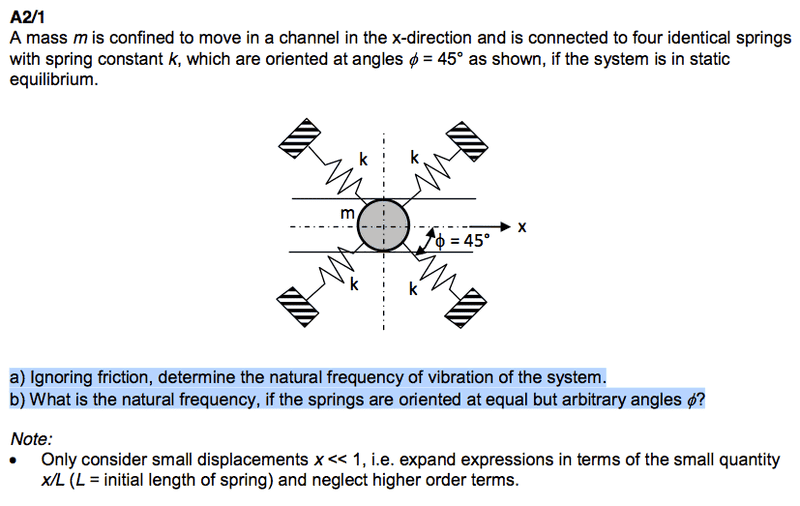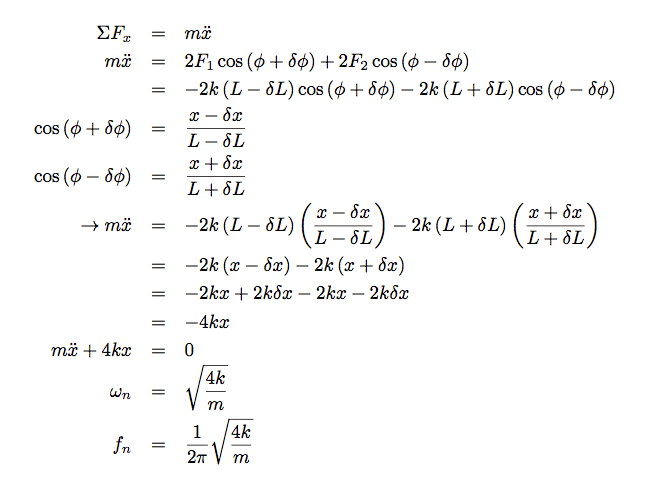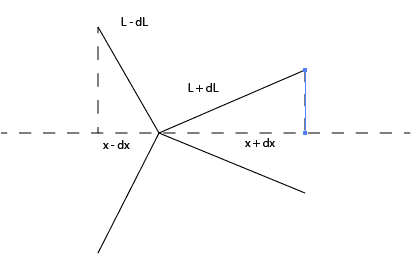# Natural Frequency of a Spring System

Malby
A mass m is confined to move in a channel in the x-direction and is connected to four identical springs with spring constant k, which are oriented at angles $\phi$ = 45° as shown, if the system is in static equilibrium.

a) Ignoring friction, determine the natural frequency of vibration of the system.
b) What is the natural frequency, if the springs are oriented at equal but arbitrary angles $\phi$?

$\omega$n = $\sqrt{\frac{k}{m}}$

Not quite sure how to go about this one. I figure you need to take a small displacement of the system to the right or less then analyse the forces such that $\Sigma$F = ma, and then derive the standard form of the spring equation from there.Malby
I worked through it again and found the natural frequency to be:

sqrt(4k/m)/(2 * Pi).

I basically let the angles on both sides change by phi +/- dphi, and the same with the length L -/+ dL, and with x. If this is the correct answer then I suppose it worked out ok.

Staff Emeritus
Homework Helper
That's the answer I would expect if Φ=0. It seems odd to me that the frequency doesn't depend on the angle at all. Do you still think that expression is correct?

Malby
That's the answer I would expect if Φ=0. It seems odd to me that the frequency doesn't depend on the angle at all. Do you still think that expression is correct?

You're right. As $\phi$ goes to 90 degrees, the natural frequency should decrease to 0, as there would be no component of force acting in the x-direction then. It appears as though multiplying by cosine would make this correct, but I don't know where that comes in in the working:In the diagram, the angle on the left is phi + dphi, the angle on the right is phi - dphi.Staff Emeritus